Question

# Find the transfer function H(s) for the circuit below. Make s=jw and plot the Bode plot...

Find the transfer function H(s) for the circuit below. Make s=jw and plot the Bode plot (magnitude and phase response) for the transfer function.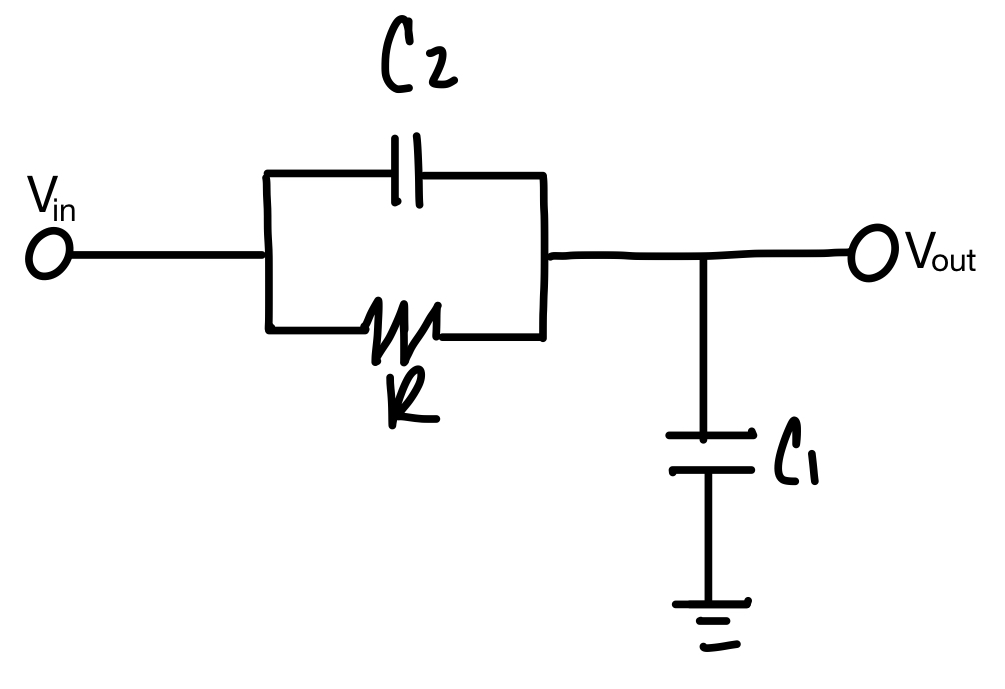C = out 페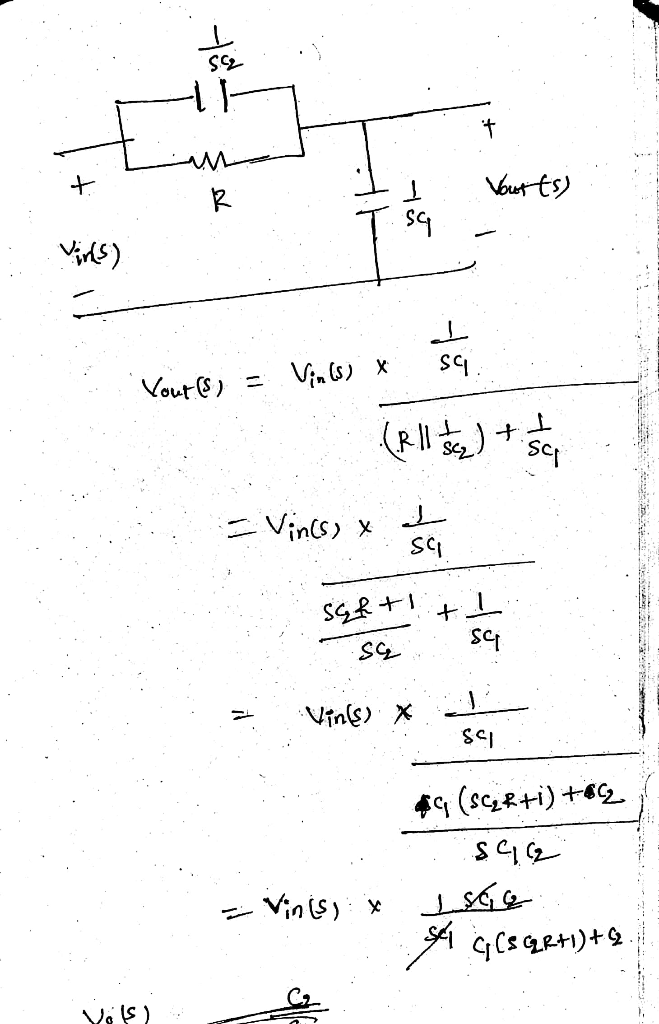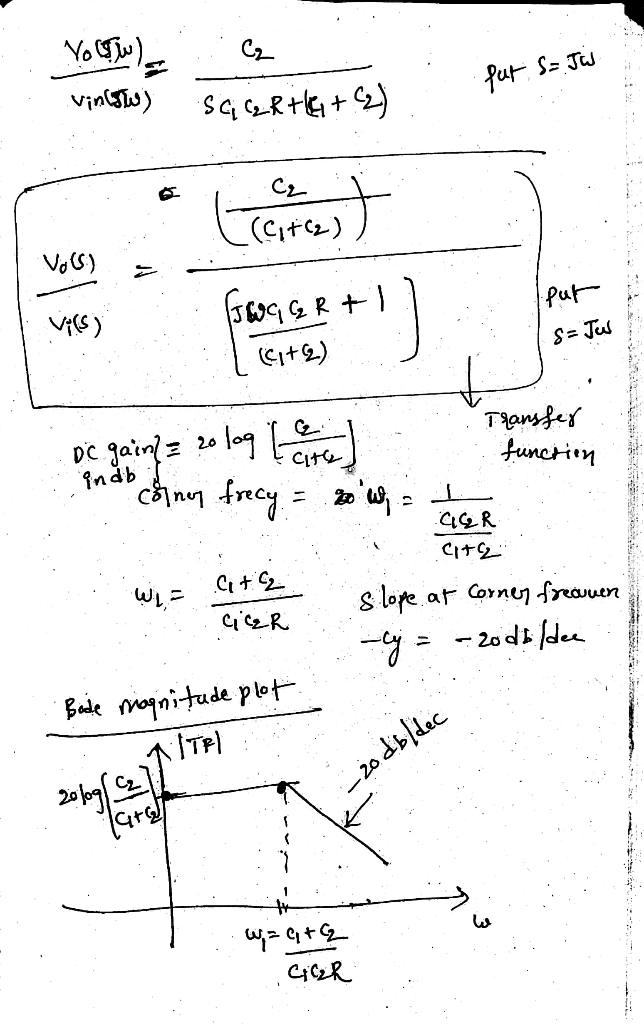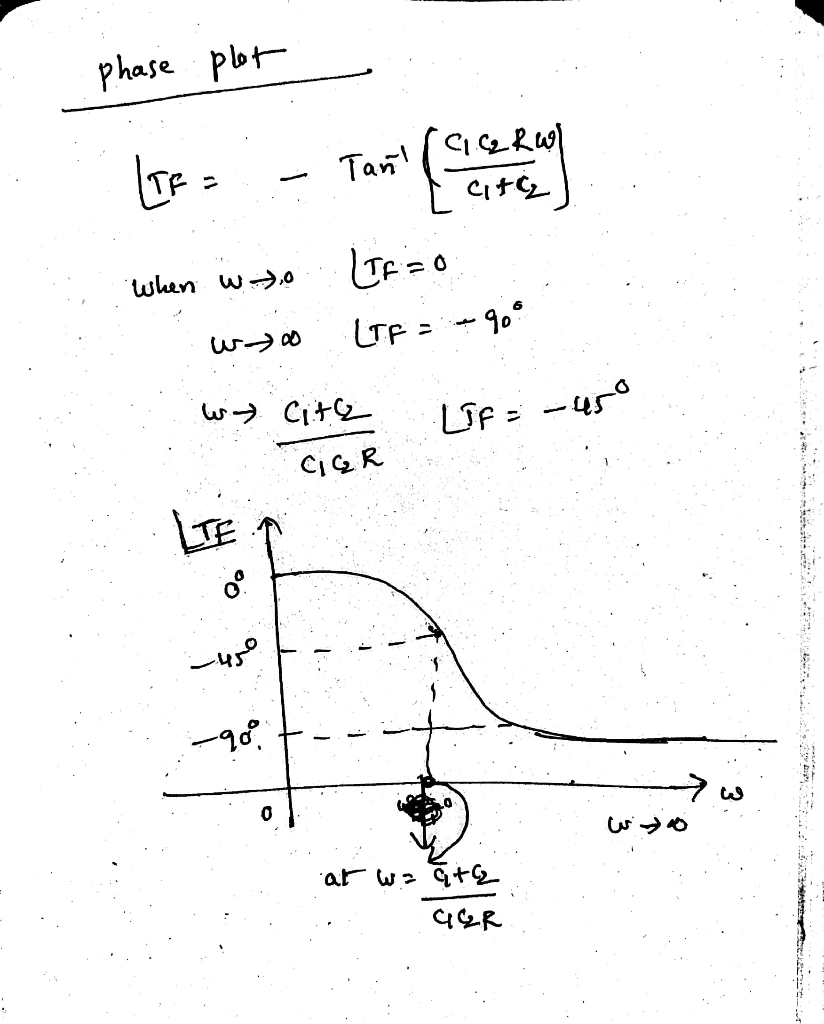#### Earn Coins

Coins can be redeemed for fabulous gifts.

Similar Homework Help Questions
• ### Find the transfer function T(jw) for the circuit below. Also find the magnitude response, 0 OP000...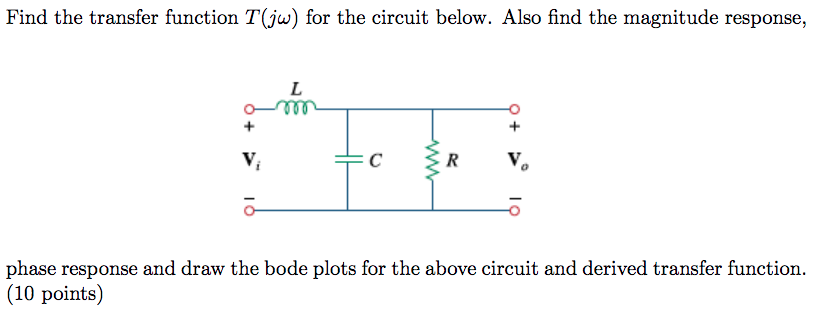Find the transfer function T(jw) for the circuit below. Also find the magnitude response, 0 OP000 + + C R 1 phase response and draw the bode plots for the above circuit and derived transfer function. (10 points)

• ### Sketch the approximate Bode plot (magnitude and phase) of the transfer function (jw) H(W) = (w...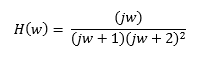Sketch the approximate Bode plot (magnitude and phase) of the transfer function (jw) H(W) = (w + 1) (w + 2)2

• ### Provide the Bode plot (sketch the gain |H(jw) and the phase shift ZH(jw)) of a system...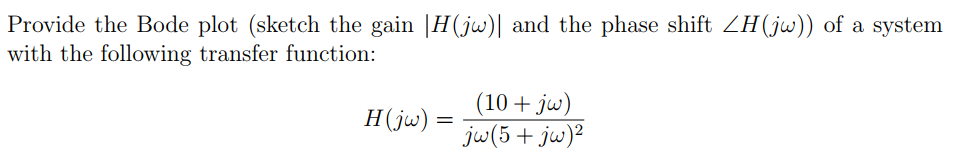Provide the Bode plot (sketch the gain |H(jw) and the phase shift ZH(jw)) of a system with the following transfer function: (10 + jw) H(jw) = jw(5 + jw)

• ### 3. Draw the Bode plot (magnitude and phase) for the transfer function H(s) of a CT...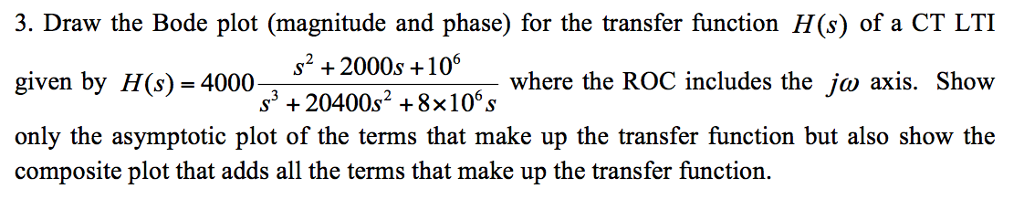3. Draw the Bode plot (magnitude and phase) for the transfer function H(s) of a CT LTI given by H(s) 4000 only the asymptotic plot of the terms that make up the transfer function but also show the composite plot that adds all the terms that make up the transfer function. S+2000s+10 where the ROC includes the ja axis. Show

• ### QUESTION #2 PLEASE 1. Derive the transfer function for the circuit shown below. Plot H(s) versus...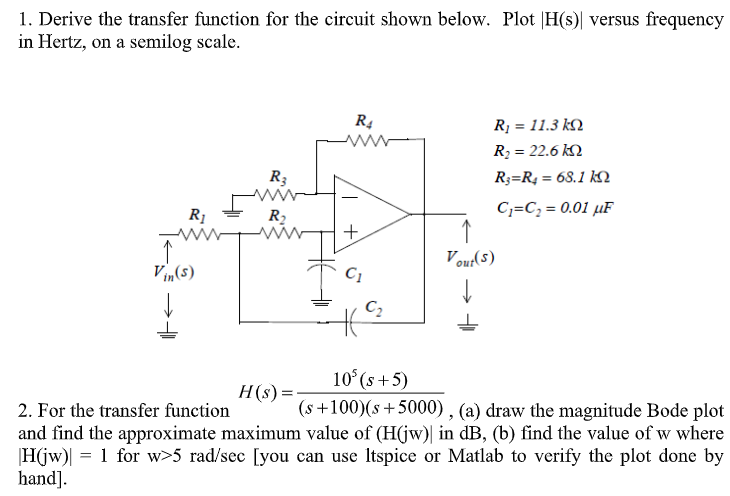QUESTION #2 PLEASE 1. Derive the transfer function for the circuit shown below. Plot H(s) versus frequency in Hertz, on a semilog scale. Ri 11.3 k Ri 22.6 k R R = 68.1 kN R3 C C 0.01 uF R2 Vout(s) Vin(s) C2 10 (s+5) H(s) = (s+100)(s5000) , (a) draw the magnitude Bode plot 2. For the transfer function and find the approximate maximum value of (H(jw) in dB, (b) find the value of w where 1 for w>5...

• ### the circuit shown, 1. Find the transfer function H(jw) 2. If R R2 12 and L1mH, plot the frequency response (both the ga...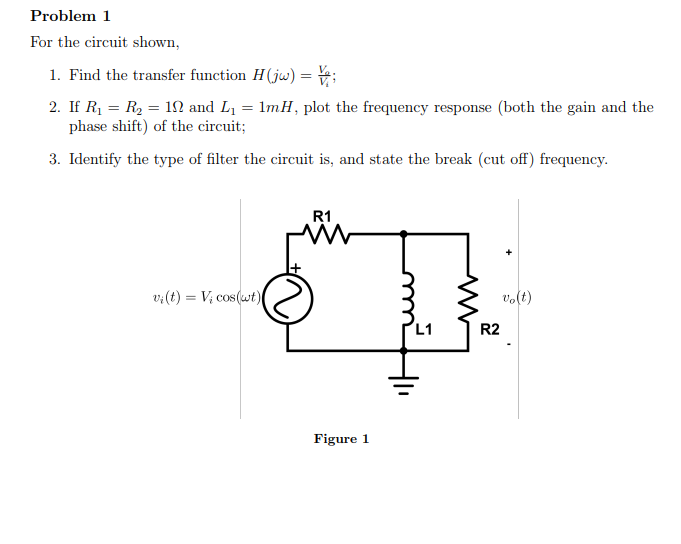the circuit shown, 1. Find the transfer function H(jw) 2. If R R2 12 and L1mH, plot the frequency response (both the gain and the phase shift) of the circuit; 3. Identify the type of filter the circuit is, and state the break (cut off) frequency. R1 v(t)Vcos(ut) L1 R2 Figure 1 the circuit shown, 1. Find the transfer function H(jw) 2. If R R2 12 and L1mH, plot the frequency response (both the gain and the phase shift) of...

• ### Problem Three: Estimating a Transfer Function from a Bode Plot Based on the Bode Plot below,...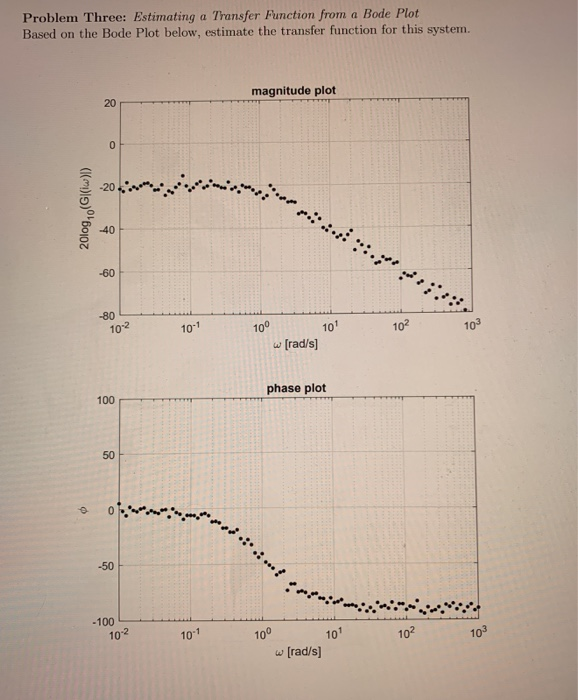Problem Three: Estimating a Transfer Function from a Bode Plot Based on the Bode Plot below, estimate the transfer function for this system. magnitude plot 20 0 2010g, ((w)) 40 -60 -80 10-2 10-1 10° 101 102 w (rad/s) phase plot 100 50 © -50 -100 102 10" 10° 102 10 101 (rad/s]

• ### Sketch the bode plot of a signal conditioner with the transfer function G(s) in the provided...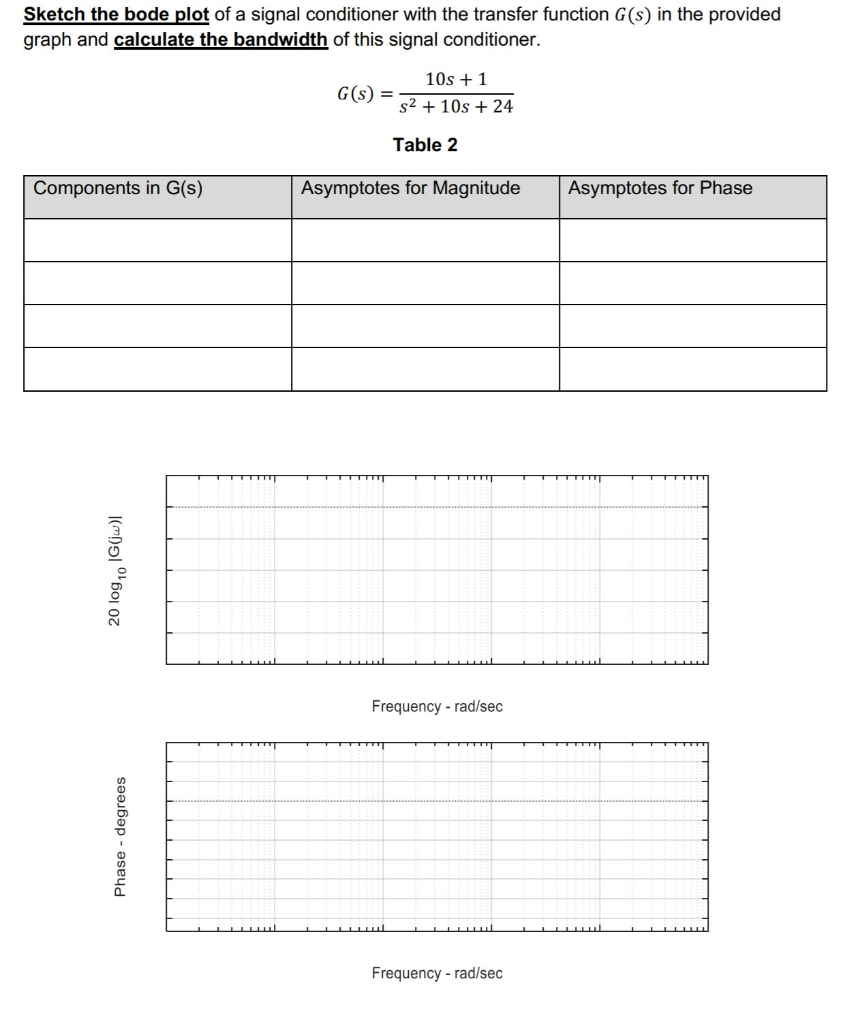Sketch the bode plot of a signal conditioner with the transfer function G(s) in the provided graph and calculate the bandwidth of this signal conditioner. GO 10s +1 S2 + 10s + 24 Table 2 Components in G(S) Asymptotes for Magnitude Asymptotes for Phase 20 log,0 1G(jw) Frequency-rad/sec Phase - degrees Frequency - rad/sec

• ### A bode plot of the transfer function, GS = - 25 \$2+45+25, is shown as below....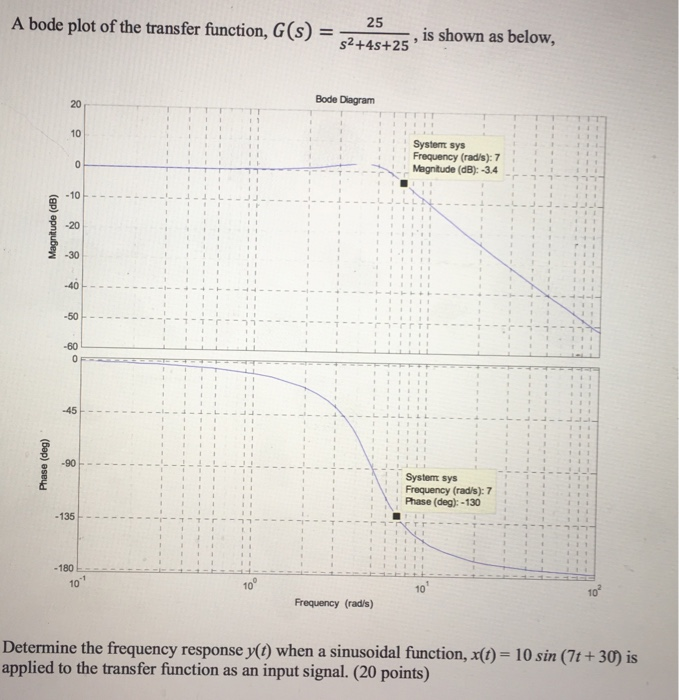A bode plot of the transfer function, GS = - 25 \$2+45+25, is shown as below. Bode Diagram System sys Frequency (rad/s): 7 Magnitude (dB): -3.4 Magnitude (dB) Phase (deg) Systemt sys Frequency (rad/s): 7 Phase (deg): - 130 - 135 - 180 10 Frequency (rad/s) Determine the frequency response y(t) when a sinusoidal function, X(t) = 10 sin (7t +30) is applied to the transfer function as an input signal. (20 points)

• ### Q11 (20pts.): For the circuit shown below Derive the transfer function, H(s) assuming the OPAMP's transfer...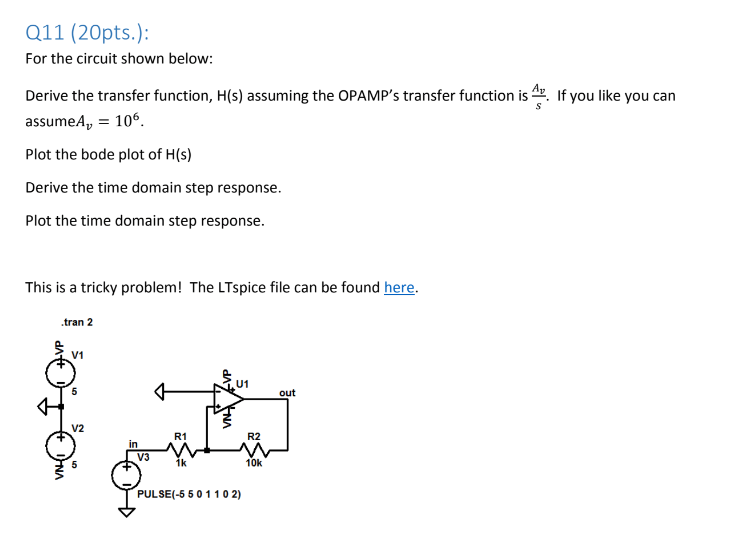Q11 (20pts.): For the circuit shown below Derive the transfer function, H(s) assuming the OPAMP's transfer function is. If you like you can assumeA 106 Plot the bode plot of H(s) Derive the time domain step response. Plot the time domain step response This is a tricky problem! The LTspice file can be found here. .tran 2 V1 U1 out V2 R1 R2 in V3 1k 10k PULSE(-5 50 1102)